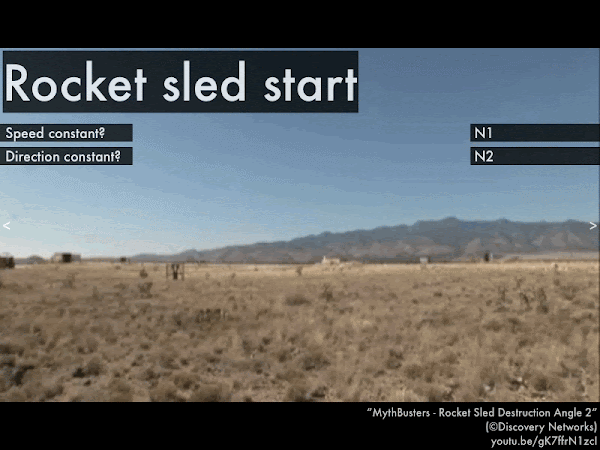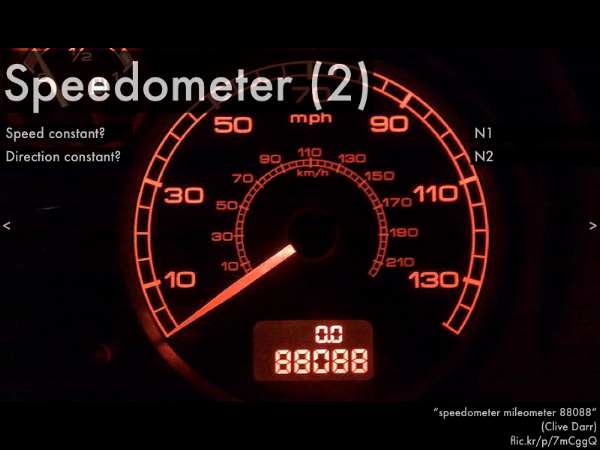## 20190909

### Online reading assignment: forces and motion

Physics 205A, fall semester 2019
Cuesta College, San Luis Obispo, CA

Students have a bi-weekly online reading assignment (hosted by SurveyMonkey.com), where they answer questions based on reading their textbook, material covered in previous lectures, opinion questions, and/or asking (anonymous) questions or making (anonymous) comments. Full credit is given for completing the online reading assignment before next week's lecture, regardless if whether their answers are correct/incorrect. Selected results/questions/comments are addressed by the instructor at the start of the following lecture.

The following questions were asked on reading textbook chapters and previewing a presentation on forces and motion.Selected/edited responses are given below.

Describe what you understand from the assigned textbook reading or presentation preview. Your description (2-3 sentences) should specifically demonstrate your level of understanding.
"I understood the motion flowcharts very well. By this, it represents when we should and when we should not use Newton's first and second law. It does this by asking whether the speed is constant and then whether the direction is constant. I understand the flowcharts and the seemingly easy two-step verification process for determining the specific Newton's law. I can observe how the flowchart could be used to decipher the correct Newton's law at play in a situation."

"I understand that in order for Newton's first law to apply, the speed and direction of an object must BOTH be constant. If either aren't, than Newton's second law applies. I also understand that Newton's first law is applicable when the net force of an object is zero, and the Newton's second law applies if the object's force is anything but zero."

"The basic postulates of Newton's first and second laws of motion. I know that the first law states that an object will continue to move at a constant velocity until an outside force causes that to change. The second law then goes on to say that acceleration of a moving object depends on the object's mass and the net force acting on the object."

"With constant direction and speed, Newton's first law is in motion; all other cases are second law. Similarly, if the net force is equal to zero, it is the first law. ΣF = ma is the equation used and can end up being 0 = 0 (for the first law case)."

"Newton's first law is applied when an object is maintaining a constant velocity; Newton's second law is applied when an object’s velocity has changed and we have to take into account the net force acted upon the object and inertia of the object."

"An object will stay at rest or in motion at a constant speed unless it is compelled to a change in net force. The net force is also the vector sum of all forces acting upon an object.

Describe what you found confusing from the assigned textbook reading or presentation preview. Your description (2-3 sentences) should specifically identify the concept(s) that you do not understand.
"I was a bit lost when viewing the net force flowchart. It seems overly simplified. But after researching it more, I am slightly more comfortable using it for the questions."

"Something I found confusing was how vectors work in Newton's second law. If you are moving in a circle in constant motion, then would the forces be considered non-zero or zero because of the changing direction?"

"Where I get confused is when I overthink and take too many things into consideration with the object. Or when I forget to take things into consideration."

"The concept of net force. Honestly the pictures in the presentation made me more confused."

"I don't see how to calculate the net force of an object when two different forces occur in different directions, as well as the other factors such as air resistance and friction. I was confused by how to determine whether Newton's first law is being used or Newton's second law. I also was a bit confused by some of the equations for the vector components of the second law."

"I am having a difficult time understanding what we are getting to in class."

Identify whether a zero or non-zero net force corresponds to Newton's first law or Newton's second law. (Only correct responses shown.)
Zero net force (ΣF = 0): Newton's first law ("N1") [92%]
Non-zero net force (ΣF ≠ 0): Newton's second law ("N2") [88%]For the rocket sled, Newton's __________ law applies to the motion of this object, and the forces acting on the object add up to a __________ net force.
 first; zero. *************  second; non-zero. ********************************  (Unsure/guessing/lost/help!) ***While the F-35B is descending at a steady rate (before it touches the ground), Newton's __________ law applies to the motion of this object, and the forces acting on the object add up to a __________ net force.
 first; zero. *****************************  second; non-zero. **************  (Unsure/guessing/lost/help!) *****For this car with a steady speedometer reading (and assuming the shaking is due to the person holding the camera, and not from the car itself), Newton's __________ law applies to the motion of this object, and the forces acting on the object add up to a __________ net force.
 first; zero. *****************************  second; non-zero. **************  (Unsure/guessing/lost/help!) *****For a person in the swinging chair ride moving along a circular trajectory at a constant speed, Newton's __________ law applies to the motion of this object, and the forces acting on the object add up to a __________ net force.
 first; zero. ******************  second; non-zero. *************************  (Unsure/guessing/lost/help!) *****For this car with a zero speedometer reading, Newton's __________ law applies to the motion of this object, and the forces acting on the object add up to a __________ net force.
 first; zero. ******************************************  second; non-zero. ****  (Unsure/guessing/lost/help!) ** 

Ask the instructor an anonymous question, or make a comment. Selected questions/comments may be discussed in class.
"Is there ever a situation when Newton's laws don't apply?" (No. Either motion is constant (Newton's first law), or is not constant (Newton's second law).)

"For the most part, this section was pretty straightforward. The only thing I would like touched upon would be the flowchart, in case I am not overthinking it. Is it as simple as it appears?" (Yes.)

Can you go over these in class with a couple of examples?"

"Will we go over the answers to the questions above in class?" (For some of these, yes.)

"Is there an answer key for these questions?" (Make sure you review these responses (with the correct answers in boldface) as part of the next reading assignment.)

"Definitely would appreciate discussion on the differences between Newton's first and second law in real physics problems."

"In the rocket sled example, is the speed constant even though the sled is accelerating when it first fires up?" (No, the speed is not constant once the rocket fires up, as it starts off stationary, and then begins to speed up, so its motion is then covered by Newton's first law (even though the direction is constant, though.)

"If an object is moving in a circular motion, is that 'constant?'" (Even if the speed is constant, the direction of the object's velocity vector is always changing, so its motion is then covered by Newton's second law.)

"What should we know about working with free body diagrams in this class?" (Nothing official, yet, but we'll get started on Wednesday.)

"what does is mean when it says forces don't 'cancel?'" (Two forces can 'cancel' in the sense that their contributions are opposite and as a result nothing happens, but it's not like they disappear or vanish--they're still there, its just that there is no net effect on the object.)

"When would vertical acceleration be negative and when would it be positive?" (If vertical acceleration is downwards/negative (say, only the force of gravity is acting on an object, and there is no air resistance), then an object moving downwards (in the direction of the net force) will speed up, and an object moving upwards will slow down. If vertical acceleration is upwards/positive (say, a rope is pulling upwards with a force greater than the weight force), then an object moving upwards (in the direction of the net force) will speed up, and an object moving downwards will slow down.)

"If Earth is spinning, how is it an inertial reference point?" (For our purposes, it's a "good enough" inertial reference frame given the situations we'll be dealing with in this course, especially in lab, where our scales, motion sensors, etc. are nowhere near sensitive enough to detect that Earth is not actually stationary. It's similar to neglecting air resistance for free fall, as that is also another "good enough" approximation to situations we'll analyze in lab.)

"Getting into forces. Things are now gonna get a little more overwhelming."

"Yee haw."